# Hurewicz map is well-defined

Jump to: navigation, search

## Statement

### Loose statement

Let$X$ be a path-connected space. For$n$ a positive integer, we want to show that the$n^{th}$ Hurewicz map based at$x_0$ of$X$ is a well-defined map: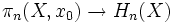$\pi_n(X,x_0) \to H_n(X)$

where$\pi_n(X,x_0)$ is the$n^{th}$ homotopy group, and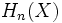$H_n(X)$ is the$n^{th}$ singular homology group.

### The strict map

The map is defined as follows. First define a map: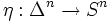$\eta:\Delta^n \to S^n$

which essentially uses the identification of$S^n$ with the quotient of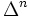$\Delta^n$ by the collapse of its boundary to a single point, i.e., a homeomorphism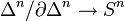$\Delta^n/\partial \Delta^n \to S^n$.

Now given any based continuous map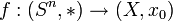$f: (S^n,*) \to (X,x_0)$, consider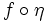$f \circ \eta$. This gives a$n$-singular chain in$X$, and its homology class is precisely the element we are looking for.

### What we need to show

To note that this is indeed well-defined, we need to show that if$f_1$ and$f_2$ are homotopic maps as based continuous maps from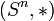$(S^n,*)$ to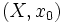$(X,x_0)$, then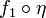$f_1 \circ \eta$ and$f_2 \circ \eta$ are both in the same homology class.

## Proof

Fill this in later